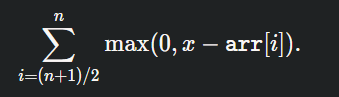# Silver: Maximum Median

Link to question: Problem - C - Codeforces

So here is what I understand so far, we can use the following formula to calculate the number of operations needed to raise the median value to x:Then all we do is try different x values and see how many operations that would take to do. So based on the answer, we use binary search to try out the different x values. Now I’m confused about how to use the binary search in this case. What is the first x value we would try? And when will the binary search loop terminate?

from the solution notes:

Then, we binary search for the maximum possible median.

Yes can you explain that binary search more. What is the first x value we would try? And when will the binary search loop terminate?

it would first have a starting point that is always correct (pos in this case). Then, it would first try pos + 2^9 since max=2^9 currently. if it worked, then it would increment pos by max. Then, it would divide max by 2. It would repeat to check if pos+max would work until max=0. the `while(check(pos+a))` is just there for boundary conditions.

1 Like

Thanks for the details. You mentioned pos initially is set to a value that is correct. What do you mean by correct, correct on what sense? Pos can’t be a correct median value, because we can’t assume 0 would always be a working median.

Secondly, when you say you do pos+max if it is correct, what do we do it doesn’t work? Do we do pos-max?

Thanks!

This link explains the solution well: Codeforces Round #577 (Div 2) Editorial - Codeforces